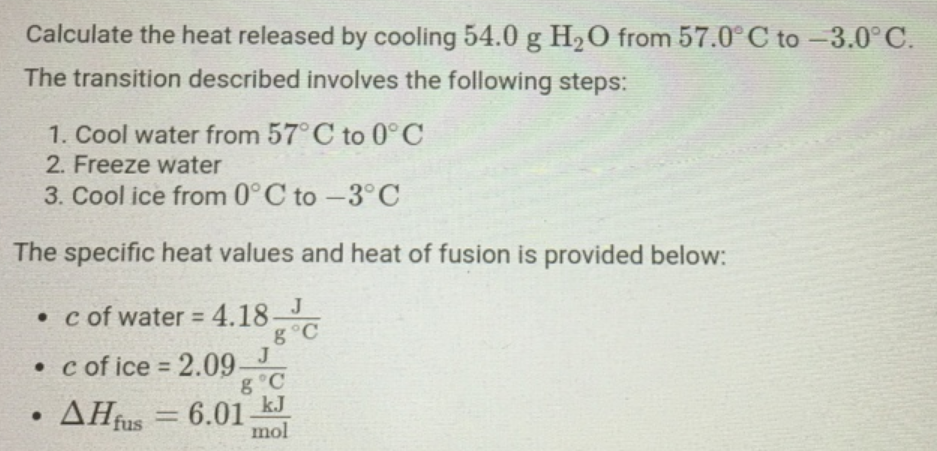# Problem: Calculate the heat released by cooling 54.0 g H2O from 57.0 C to -3.0 C. The transition described involves the following steps:1. Cool water from 57  C to 0 C2. Freeze water3. Cool ice from 0 C to -3  CThe specific heat values and heat of fusion is provided below: c of water = 4.18 J/g degree C c of ice = 2.09 J/g degree C Delta Hfus = 6.01 kJ/mol

🤓 Based on our data, we think this question is relevant for Professor Ahuja's class at GSU.

###### FREE Expert Solution###### Problem Details

Calculate the heat released by cooling 54.0 g H2O from 57.0 C to -3.0 C. The transition described involves the following steps:

1. Cool water from 57  C to 0 C
2. Freeze water
3. Cool ice from 0 C to -3  C

The specific heat values and heat of fusion is provided below: c of water = 4.18 J/g degree C c of ice = 2.09 J/g degree C Delta Hfus = 6.01 kJ/mol